Next: The Orbits Up: Equations of Motion Previous: Equations of Motion

## The Lagrangian

The main parts of the deduction in this subsection is taken from [Chapter 7]P-80.

As seen in the previous section, the Lagrangian is defined as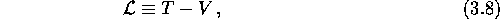where T is the kinetic energy and V is the potential energy. In physical coordinates we have, for one particle,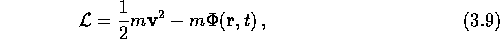whereis the potential. Since we in this treatment are considering comoving particles, we need to transform equation (3.9) to comoving coordinates, using equations (2.5) and (2.6):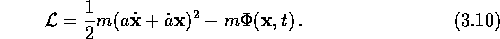This expression can be simplified somewhat by adding a function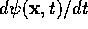, which will cancel out some of its terms. This seems like a rather dubious thing to do, but is justified by Hamilton's principle: The time integral of the functiononly depends on the values of its variables at the end-points, where the variation is zero. Therefore, the function does not contribute to the time integral of the Lagrangian. The function added in this case is given by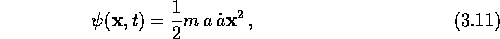which, by subtracting its time derivate from equation (3.10),results in the reduced Lagrangian: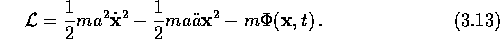The second term in equation (3.13) allows the introduction of a homogeneous mass distribution, which in this case would represent the background universe. The Friedmann equations for a flat, pressureless universe is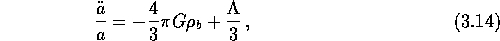whereis the mean background density. Inserting this equation into (3.13), prompts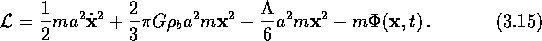The second term is the result of the coordinate change from physical to comoving, and can be considered as a correction term to the potentialdue to the background universe.

Before looking closer at what the expression for the potential is, we need to include more particles than the one treated so far. The reason for this is that in the approximation considered here, the only potential affecting a particle is the one formed by the gravitational field of the other particles in the system. Hence, the Lagrangian for a system of particles is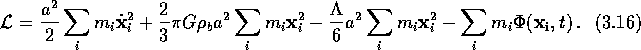The potential energyin equation (3.16) can be derived from Poisson's equation (which in turn can be derived from the Newtonian approximation to general relativity). Poisson's equation with p=0 and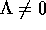is given aswhere the ``'' subscript indicates that the gradient is to be taken with respect to the comoving coordinate. The solution of this equation for one particle can easily be seen to be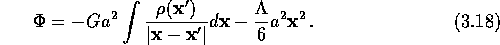For the point particle picture considered here, where a particle at positionis being affected by the particlesand where, the potential can be written as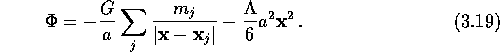By introducing this expression for the potential to equation (3.16), the terms containing the cosmological constant cancels out, and we then have the following resulting expression for the Lagrangian, describing a system of particles in an expanding universe:Next: The Orbits Up: Equations of Motion Previous: Equations of Motion

Trond Hjorteland
Mon Jul 5 02:59:28 MET DST 1999